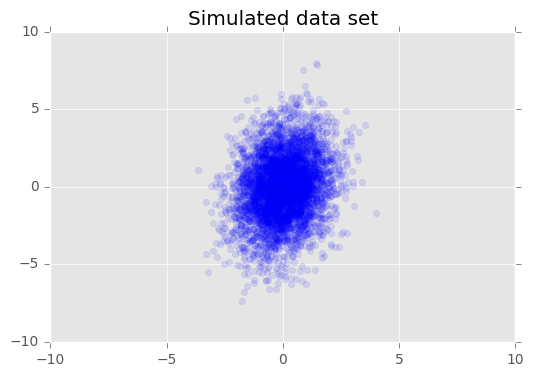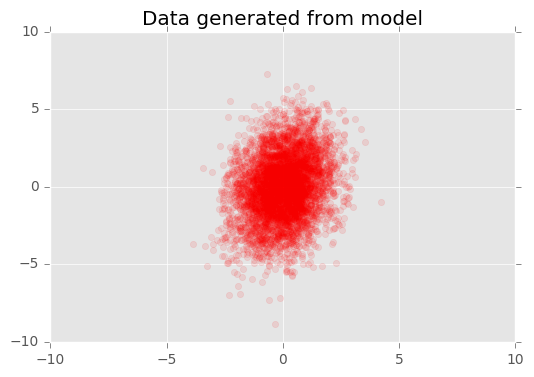## Probabilistic PCA

Probabilistic principal components analysis (PCA) is a dimensionality reduction technique that analyzes data via a lower dimensional latent space (Tipping & Bishop, 1999). It is often used when there are missing values in the data or for multidimensional scaling.

We demonstrate with an example in Edward. An interactive version with Jupyter notebook is available here.

### Data

We use simulated data. We’ll talk about the individual variables and what they stand for in the next section. For this example, each data point is 2-dimensional, $$\mathbf{x}_n\in\mathbb{R}^2$$.

def build_toy_dataset(N, D, K, sigma=1):
x_train = np.zeros((D, N))
w = np.random.normal(0.0, 2.0, size=(D, K))
z = np.random.normal(0.0, 1.0, size=(K, N))
mean = np.dot(w, z)
for d in range(D):
for n in range(N):
x_train[d, n] = np.random.normal(mean[d, n], sigma)

print("True principal axes:")
print(w)
return x_train

N = 5000  # number of data points
D = 2  # data dimensionality
K = 1  # latent dimensionality

x_train = build_toy_dataset(N, D, K)
## True principal axes:
## [[ 0.25947927]
##  [ 1.80472372]]

We visualize the data set.

plt.scatter(x_train[0, :], x_train[1, :], color='blue', alpha=0.1)
plt.axis([-10, 10, -10, 10])
plt.title("Simulated data set")
plt.show()### Model

Consider a data set $$\mathbf{X} = \{\mathbf{x}_n\}$$ of $$N$$ data points, where each data point is $$D$$-dimensional, $$\mathbf{x}_n \in \mathbb{R}^D$$. We aim to represent each $$\mathbf{x}_n$$ under a latent variable $$\mathbf{z}_n \in \mathbb{R}^K$$ with lower dimension, $$K < D$$. The set of principal axes $$\mathbf{W}$$ relates the latent variables to the data.

Specifically, we assume that each latent variable is normally distributed, $\mathbf{z}_n \sim N(\mathbf{0}, \mathbf{I}).$ The corresponding data point is generated via a projection, $\mathbf{x}_n \mid \mathbf{z}_n \sim N(\mathbf{W}\mathbf{z}_n, \sigma^2\mathbf{I}),$ where the matrix $$\mathbf{W}\in\mathbb{R}^{D\times K}$$ are known as the principal axes. In probabilistic PCA, we are typically interested in estimating the principal axes $$\mathbf{W}$$ and the noise term $$\sigma^2$$.

Probabilistic PCA generalizes classical PCA. Marginalizing out the the latent variable, the distribution of each data point is $\mathbf{x}_n \sim N(\mathbf{0}, \mathbf{W}\mathbf{W}^Y + \sigma^2\mathbf{I}).$ Classical PCA is the specific case of probabilistic PCA when the covariance of the noise becomes infinitesimally small, $$\sigma^2 \to 0$$.

We set up our model below. In our analysis, we fix $$\sigma=2.0$$, and instead of point estimating $$\mathbf{W}$$ as a model parameter, we place a prior over it in order to infer a distribution over principal axes.

from edward.models import Normal

w = Normal(loc=tf.zeros([D, K]), scale=2.0 * tf.ones([D, K]))
z = Normal(loc=tf.zeros([N, K]), scale=tf.ones([N, K]))
x = Normal(loc=tf.matmul(w, z, transpose_b=True), scale=tf.ones([D, N]))

### Inference

The posterior distribution over the principal axes $$\mathbf{W}$$ cannot be analytically determined. Below, we set up our inference variables and then run a chosen algorithm to infer $$\mathbf{W}$$. Below we use variational inference to minimize the $$\text{KL}(q\|p)$$ divergence measure.

qw = Normal(loc=tf.get_variable("qw/loc", [D, K]),
scale=tf.nn.softplus(tf.get_variable("qw/scale", [D, K])))
qz = Normal(loc=tf.get_variable("qz/loc", [N, K]),
scale=tf.nn.softplus(tf.get_variable("qz/scale", [N, K])))

inference = ed.KLqp({w: qw, z: qz}, data={x: x_train})
inference.run(n_iter=500, n_print=100, n_samples=10)

### Criticism

To check our inferences, we first inspect the model’s learned principal axes.

sess = ed.get_session()
print("Inferred principal axes:")
print(sess.run(qw.mean()))
## Inferred principal axes:
## [[-0.24093632]
##  [-1.76468039]]

The model has recovered the true principal axes up to finite data and also up to identifiability (there’s a symmetry in the parameterization).

Another way to criticize the model is to visualize the observed data against data generated from our fitted model.

x_post = ed.copy(x, {w: qw, z: qz})
x_gen = sess.run(x_post)

plt.scatter(x_gen[0, :], x_gen[1, :], color='red', alpha=0.1)
plt.axis([-10, 10, -10, 10])
plt.title("Data generated from model")
plt.show()The generated data looks close to the true data.

### Acknowledgments

We thank Mayank Agrawal for writing the initial version of this tutorial.

Tipping, M. E., & Bishop, C. M. (1999). Probabilistic principal component analysis. Journal of the Royal Statistical Society: Series B (Statistical Methodology), 61(3), 611–622.